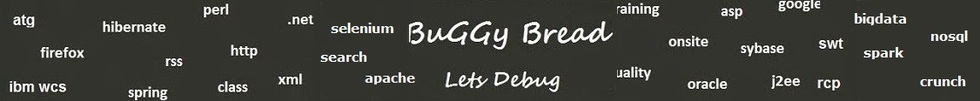## Search Java Classes and Packages

Search Java Frameworks and Libraries

255581 classes and counting ...
 Search Tips Index Status

#Com.vividsolutions.jts.algorithm Classes and Interfaces - 17 results found.
 Name Description Type Package Framework Angle Utility functions for working with angles. Class com.vividsolutions.jts.algorithm JTS Topology BoundaryNodeRule An interface for rules which determine whether node points which are in boundaries of lineal geometry components Interface com.vividsolutions.jts.algorithm JTS Topology BoundaryNodeRule .EndPointBoundaryNodeRule A BoundaryNodeRule which specifies that any points which are endpoints of lineal components are in the boundary of the Class com.vividsolutions.jts.algorithm JTS Topology BoundaryNodeRule .Mod2BoundaryNodeRule A BoundaryNodeRule specifies that points are in the boundary of a lineal geometry iff Class com.vividsolutions.jts.algorithm JTS Topology BoundaryNodeRule .MonoValentEndPointBoundaryNodeRule A BoundaryNodeRule which determines that only endpoints with valency of exactly 1 are on the boundary. Class com.vividsolutions.jts.algorithm JTS Topology BoundaryNodeRule .MultiValentEndPointBoundaryNodeRule A BoundaryNodeRule which determines that only endpoints with valency greater than 1 are on the boundary. Class com.vividsolutions.jts.algorithm JTS Topology CGAlgorithms Specifies and implements various fundamental Computational Geometric algorithms. Class com.vividsolutions.jts.algorithm JTS Topology CentralEndpointIntersector Computes an approximate intersection of two line segments by taking the most central of the endpoints of the segments. Class com.vividsolutions.jts.algorithm JTS Topology CentroidArea Computes the centroid of an area geometry. Class com.vividsolutions.jts.algorithm JTS Topology CentroidLine Computes the centroid of a linear geometry. Class com.vividsolutions.jts.algorithm JTS Topology CentroidPoint Computes the centroid of a point geometry. Class com.vividsolutions.jts.algorithm JTS Topology ConvexHull Computes the convex hull of a Geometry. Class com.vividsolutions.jts.algorithm JTS Topology HCoordinate Represents a homogeneous coordinate in a 2-D coordinate space. Class com.vividsolutions.jts.algorithm JTS Topology InteriorPointArea Computes a point in the interior of an area geometry. Class com.vividsolutions.jts.algorithm JTS Topology InteriorPointLine Computes a point in the interior of an linear geometry. Class com.vividsolutions.jts.algorithm JTS Topology InteriorPointPoint Computes a point in the interior of an point geometry. Class com.vividsolutions.jts.algorithm JTS Topology LineIntersector A LineIntersector is an algorithm that can both test whether two line segments intersect and compute the intersection point Class com.vividsolutions.jts.algorithm JTS Topology